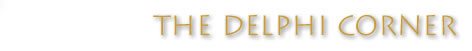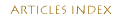Pulling Digits Out of String to Sum Them

How do I pull out every second digit from a string and sum them?

This is a rather unusual question, but it's not that hard to accomplish.

What must iterate through each character of the string and "grab" the digits whose position is a multiple of two (2). There are a couple of ways to do this, but I took what I felt was the easier route. Since this is more or less a binary problem, setting a Boolean value with each iteration works nicely. Here's the logic:

1. For all "odd" positions, set Boolean value to False;
2. For all "even" positions, set Boolean value to True;
3. If the Boolean value is true, grab that character and add it to a temporary buffer.
4. Iterate through the buffer, and convert each character to an Integer while adding the converted value to an integer variable.

Here's the code that accomplishes the above:

```function AddEvenOrOddChars(S : String; OddOrEven : Boolean) : Integer;
var
I   : Integer;
evn : Boolean;
buf : String;
begin
Result := 0;

{If OddOrEven was passed as True, then the all odd positions
will be grabbed and summed. If False, then all even positions
will be grabbed and summed.}
evn := EvenOdd;

{First grab the even position characters}
for I := 1 to Length(S) do begin
if evn then
buf := buf + S[I];

{Set boolean to its opposite regardless of its current value.
If it's currently true, then we've just grabbed a character.
Setting it to False will make the program skip the next one.}
evn := NOT evn;
end;

{Now, iterate through the buffer variable to add up the individual
values}
for I := 1 to Length(buf) do
Result := Result + StrToInt(buf[I]);
end;```